Bihar Board 12th Physics Objective Questions and Answers

## Bihar Board 12th Physics Objective Answers Chapter 4 Moving Charges and Magnetism

Question 1.
A strong magnetic Held is applied on a stationary electron. Then the electron.
(a) moves in the direction of the field.
(b) remains stationary.
(c) moves perpendicular to the direction of the field.
(d) move opposite to the direction of the field.
(b) remains stationary.Question 2.
The magnetic force $$\vec{F}$$ on a current carry ing conductor of length l in an external magnetic Field $$\vec{B}$$ is give by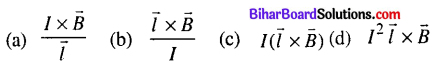(c) $$I(\vec{l} \times \bar{B})$$

Question 3.
A circular loop of radius R carrying a current I is placed in a uniform magnetic field B perpendicular to the loop. The force on the loop is
(a) 2πRIB
(b) 2πRI2B2
(c) πR2IB
(d) zero
(d) zero

Question 4.
The magnetic force per unit length on a wire carrying a current of 10 A and making an angle of 45° with the direction of a uniform magnetic field of 0.20 T is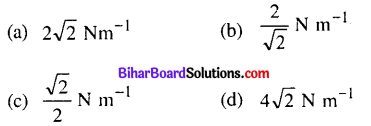(b) $$\frac{2}{\sqrt{2}} \mathrm{N} \mathrm{m}^{-1}$$
Solution:
l = 10A,θ = 45°,R = 0.2T
∴F = IlB sin θ
$$\frac{F}{l}=I B \sin 45^{\circ}=10 \times 0.2 \times \frac{1}{\sqrt{2}}=\frac{2}{\sqrt{2}} \mathrm{N} \mathrm{m}^{-1}$$Question 5.
An electron of energy 1800 eV describes a circular path in magnetic field of flux density 0.4 T. The radius of path is (q = 1.6 × 10-19 C, me = 9.1 × 10-31 kg)
(a) 2.58 × 10-4 m
(b) 3.58 × 10-4 m
(c) 2.58 × 10-3 m
(d) 3.58 × 10-3 m
(b) 3.58 × 10-4 m
Solution: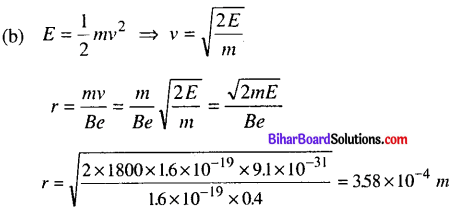Question 6.
Two α-particles have the ratio of their velocities as 3 : 2 on entering the field. If they move in different circular paths, then the ratio of the radii of their paths is
(a) 2 : 3
(b) 3 : 2
(c) 9 : 4
(d) 4 : 9
(b) 3 : 2
Solution: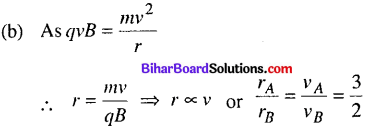Question 7.
A charged particle would continue to move with a constant velocity in a region wherein, which of the following conditions is not corrent ?
(a) E = 0, B ≠ 0
(b) E ≠ 0, B ≠ 0
(c) E ≠ 0, B = 0
(d) E = 0, B = 0
(c) E ≠ 0, B = 0Question 8.
The cyclotron frequency vc. is given by(a) $$\frac{q B}{2 \pi m}$$

Question 9.
A charged particle is moving in a cyclotron, what effect on the radius of path of this charged particle will occur when the frequency of the radio frequency field is doubled ?
(a) It will also be doubled.
(b) It will be halved.
(c) It will be increased by four times.
(d) It will remain unchanged.
(d) It will remain unchanged.

Question 10.
If an electron is moving in a magnetic field of 5.4 v 10-4 T on a circular path of radius 32 cm having a fequency of 2.5 MHz, then its speed will be
(a) 8.56 × 106 m s-1
(b) 5.024 × 106 m s-1
(c) 8.56 × 104 m s-1
(d) 5.024 × 104 m s-1
(b) 5.024 × 106 m s-1
Solution:
(b) Here, B = 5.4 × 10-4T;
r = 32cm = 32 × 10-2m v = 2.5 MHz = 2.5 × 106Hz
The speed of electron on circular path
v = r × 2πυ) = 32 × 10-2 × 2 x 3.14 × 2.5 × 106
= 502.4 × 104 = 5.024 × 106 m s-1

Question 11.
A proton and an a-particle enter in a uniform magnetic field perpendicularly with same speed. The ratio of time periods of both particle
$$\left(\frac{T_{p}}{T_{\alpha}}\right)$$ will be
(a) 1 : 2
(b) 1 : 3
(c) 2 : 1
(d) 3 : 1
(a) 1 : 2

Question 12.
A proton, a deutron and an a-particle with same kinetic energy enter perpendicularly in a uniform magnetic Held, then the ratio of radii of their ciruclar paths is
(a) 1 : 1 : √2
(b) √2 : 1 : 1
(c) 1 : √2 : 1
(d) 1 : 2 : √2
(c) 1 : √2 : 1Question 13.
The energy of emergent protons in MeV from a cyclotron having radius of its dees 1.8 m and applied magnetic Field 0.7 T is (mass of proton = 1.67 × 10-27 kg)
(a) 50
(b) 60
(c) 70
(d) 76
(d) 76
Solution: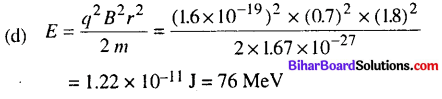Question 14.
The operating magnetic field for accelerating protons in a cyclotron oscillator having frequency of 12 MHz is (q = 1.6 × 10-19 C, mp = 1.67 × 10-27 kg and 1 MeV = 1.6 × 10-13 J)
(a) 0.69 T
(b) 0.79 T
(c) 0.59 T
(d) 0.49 T
Solution:
(b) 0.79 T
(b)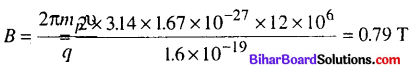Question 15.
A circular loop of radius 3 cm is having a current of 12.5 A. The magnitude of magnetic field at a distance of 4 cm on its axis is
(a) 5.65 × 10-5 T
(b) 5.27 × 10-5 T
(c) 6.54 × 10-5 T
(d) 9.20 × 10-5 T
(a) 5.65 × 10-5 T
Solution: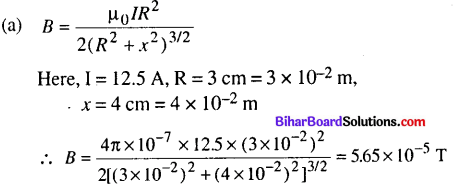Question 16.
A circular coil of wire consisting of 100 turns each of radius 9 cm carries a current of 0.4 A. The magnitude of magnetic field at the centre of the coil is
(a) 2.4 × 10-4 T
(b) 3.5 × 10-4T
(c) 2.79 × 10-4
(d) 3 × 10-4
(c) 2.79 × 10-4
Solution: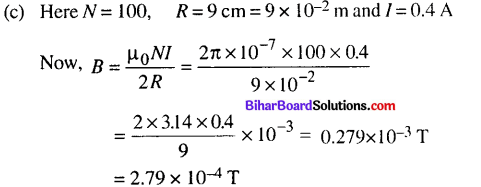Question 17.
The magnitude of the magnetic field at the centre of the tightly wound 150 turn coil of radius 12 cm carrying a current of 2 A is
(a) 18 G
(b) 19.7 G
(c) 15.7 G
(d) 17.7 G
(c) 15.7 G
Solution: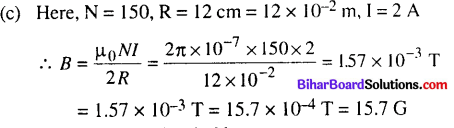Question 18.
A tightly wound 90 turn coil of radius 15 cm has a magnetic field of 4 × 10-4 T at its centre. The current flowing through it is
(a) 1.06 A
(b) 2.44 A
(c) 3.44 A
(d) 4.44 A
(a) 1.06 AQuestion 19.
Ampere’s circuital law is given by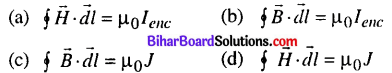(b) $$\oint \vec{B} \cdot \overrightarrow{d l}=\mu_{0} I_{e n c}$$

Question 20.
A long straight wire in the horizontal plane carries a current of 75 A in north to south direction, magnitude and direction of field B at a point 3 m east of the wire is
(a) 4 × 10-6 T, vertical up
(b) 5 × 10-6 T, vertical down
(c) 5 × 10-6 T, vertical up
(d) 4 × 10-6 T, vertical down
(c) 5 × 10-6 T, vertical up
Solution:
From Ampere circuital lawThe direction of field at the given point will be vertical up determined by the screw rule or right hand rule.

Question 21.
The nature of parallel and anti-parallel currents are
(a) parallel currents repel and antiparallel currents attract.
(b) parallel currents attract and antiparallel currents repel.
(c) both currents attract
(d) both currents repel.
(a) parallel currents repel and antiparallel currents attract.

Question 22.
Two parallel wires 2 m apart carry currents of 2 A and 5 A respectively in same direction, the force per unit length acting between these two wires is
(a) 2 × 10-6 N m-1
(b) 3 × 10-6 N m-1
(c) 1 × 10-6 N m-1
(d) 4 × 10-6 N m-1
(c) 1 × 10-6 N m-1
Solution: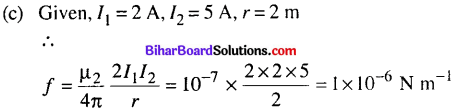Question 23.
The magnetic moment of a current / carrying circular coil of radius r and number of turns A varies as
(a) 1/
(b) 1/rr2
(c) r
(d) r2
(d) r2

Question 24.
A 200 turn closely wound circular coil of radius 15 cm carries a current of 4 A. The magnetic moment of this coil is
(a) 36.5 Am2
(b) 56.5 A m2
(c) 66.5 A m2
(d) 108 A m2
(b) 56.5 A m2
Solution:
(b) The magnetic moment is given by
|\vec{m}| = NlA = Nlπ2 = 200 × 4 × 3.14 × (15 × 10-2)2
= 200 × 4 × 3.14 × 15 × 15 × 10-4 = 56.5 A m2

Question 25.
The magnetic moment associated with a circular coil of 35 turns and radius 25 cm, if it carries a current of 11 A is
(a) 72.2 Am2
(b) 70.5 A m2
(c) 74.56 A m2
(d) 75.56 A m2
(d) 75.56 A m2
Solution:
(d) Given N = 35, r = 25 cm = 25 × 10-2 m, l = 11
A Then magnetic moment associated with this circular coil
M = NIA= NIπr2 = 35 × 11 × 3.14 x (25 × 10-2)2 = 75.56 Am2
= 75.56Am2Question 26.
Magnetic field at the centre of a circular loop of area A is B. The magnetic moment of the loop is(d) $$\frac{2 B A \sqrt{A}}{\mu_{0} \sqrt{\pi}}$$

Question 27.
A circular coil of radius 10 cm having 100 turns carries a current of 3.2 A. The magnetic field at the centre of the coil is
(a) 2.01 × 10-3 T
(b) 5.64 × 10-3 T
(c) 2.64 × 10-4 T
(d) 5.64 × 10-4 T
(a) 2.01 × 10-3 T
Solution: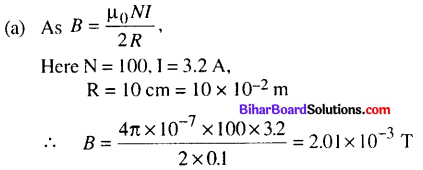Question 28.
A current carrying loop is placed in a uniform magnetic field. The torque acting on it does not depend upon
(a) area of loop
(b) value of current
(c) magnetic field
(d) None of these
(d) None of these

Question 29.
The gyromagnetic ratio of an electron in soidum atom is
(a) depending upon the atomic number of the atom
(b) depending upon the shell number of the atom
(c) independent of that orbit it is in
(d) having positive value
(c) independent of that orbit it is inQuestion 30.
Gyromagnetic ratio is the ratio of magnetic moment (μl) to the orbital angular momentum (l). Its numerical value for an electron is given by
(a) 8.8 × 10-12 C kg-1
(b) 8.8 × 1010 C kg-1
(c) 1.6 × 10-19 C kg-1
(d) 6.67 × 1011 C kg-1
(b) 8.8 × 1010 C kg-1

Question 31.
What is the correct value of Bohr magneton ?
(a) 8.99 × 10-24 A m2
(b) 9.27 × 10-24 A m2
(c) 5.66 × 10-24 A m2
(d) 9.27 × 10-28 A m2
(b) 9.27 × 10-24 A m2Question 32.
In a moving coil galvanometer the deflection (Φ) on the scale by a pointer attached to the spring is(c) $$\left(\frac{N A B}{k}\right) I$$

Question 33.
A galvanometer of resistance 70 £2 , is converted to an ammeter by a shunt resistance rs = 0.03 £2 . The value of its resistance will become
(a) 0.025 Ω
(b) 0.022 Ω
(c) 0.035 Ω
(d) 0.030 Ω
(d) 0.030 ΩQuestion 34.
In the given circuit, a galvanometer with a resistance of 70 Ω is conveted to an ammeter by a shunt resistance of 0.05 Ω , total current measured by this device is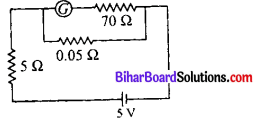(a) 0.88 A
(b) 0.77 A
(c) 0.55 A
(d) 0.99 A
(d) 0.99 A
Solution:
(d)∴ Rs = 0.0499Ω
= 0.05Ω
The total resistance in the circuit
R = Rs + 5Ω
= 0.05 + 5
= 5.05 Ω
The curent measured by the deviceQuestion 35.
The conversion of a moving coil galvanometer into a voltmeter is done by
(a) introducing a resistance of large value in series
(b) introducing a resistance of small value in parallel
(c) introducing a resistance of large value in parallel
(d) inroducing a resistance of small value is series
(a) introducing a resistance of large value in seriesQuestion 36.
A galvanometer of resistance 10 Ω gives full-scale deflection when 1 mA current passes through it. The resistance required to convert it into a voltmeter reading upto 2.5 V is
(a) 24.9 Ω
(b) 249 Ω
(c) 2490 Ω
(d) 24900 Ω
(c) 2490 Ω
Solution:Question 37.
The value of current in the given circuit if the ammeter is a galvanometer with a resistance RG = 50 Ω is(a) 0.048 A
(b) 0.023 A
(c) 0.061 A
(d) 0.094 A
(d) 0.094 A

Question 38.
The range of voltmeter of resistance 300 £2 is 5 V. The resistance to be connected to convert it into an ammeter of range 5 A is
(a) 1 Ω in series
(b) 1 Ω in parallel
(c) 0.1 Ω in series
(d) 0.1 Ω in parallel
(b) 1 Ω in parallel

Question 39.
Biot-Savart law indicates that the moving electrons (velocity $$\vec{V}$$ ) produce a magnetic field $$\vec{B}$$ such that
(a) $$\vec{B} \perp \vec{v}$$
(b) $$\vec{B} \| \vec{v}$$
(c) it obeys inverse cube law.
(d) it is along the line joining the electron and point of observation.
(a) $$\vec{B} \perp \vec{v}$$